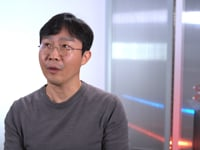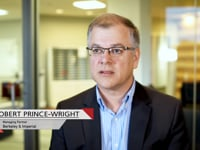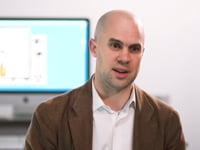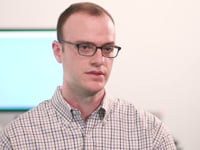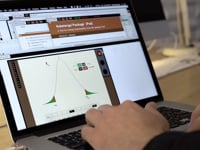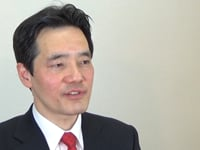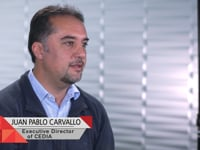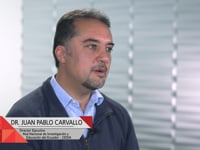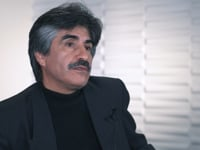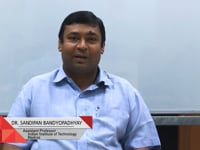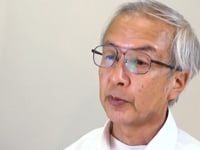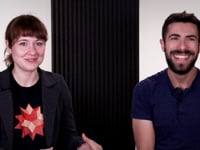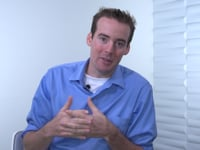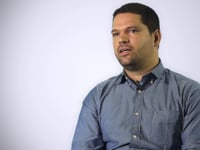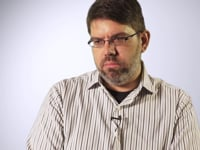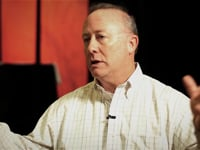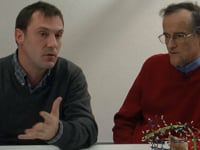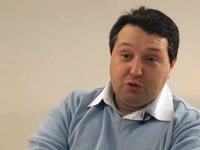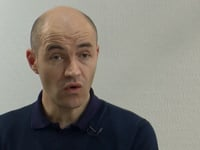Learn how »

# MATLAB to Mathematica: An Engineering Student's Perspective

UCSB electrical and computer engineering graduate student Justin Pearson shows how engineering equations can be both simplified and physically insightful using the Wolfram Language as compared to procedures in MATLAB.